# Reading 43: Portfolio Risk and Return (P.2)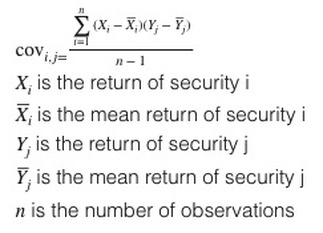• Correlation coefficient: ∈ (-1;1)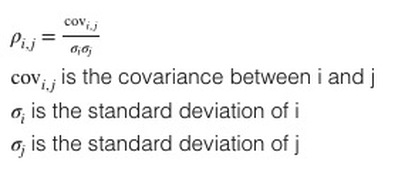? = 1: deviations from the mean or expected return are always proportional in the same direction. They are perfectly positive correlated.

? =- 1: in opposite direction. Negative correlated.

? = 0: no linear relationship between 2 stock’s return. Uncorrelated.

4. Explain risk-aversion and its implications for portfolio selection

• A risk-averse investor is simply one that dislike risk (prefer less risk than more risk). Given 2 investments that have equal expected return, risk-averse choose the less risk.
• A risk-seeking (risk-loving) prefers more risk. Given 2 investments that have equal expected return, risk-seeking will choose the more risky investment.
• A risk-neutral  investor has no preference regarding risk and would be indifferent between two such investments.

If expected returns are identical, a risk-averse investor choose least risk. However, a risk-averse investor may select a vary portfolio if he fill extra return for additional risk.

5. Calculate and interpret portfolio standard deviation

The variance of returns for a portfolio of 2 risky assets.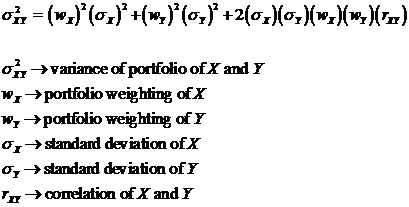The variance of returns for a portfolio of 2 risky assets.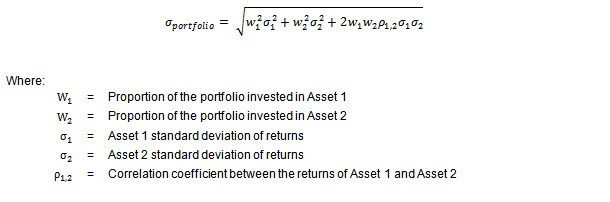6. Describe the effect on a portfolio’s risk of investing in assets that are less than perfectly correlated

(? <1)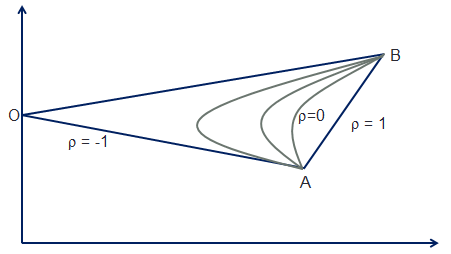Lower the correlation of asset returns ⇒ The greater the risk-reduction benefit of combination 2 assets.

The desire to reduce risk is what drives investors to invest in not just domestic stocks, but also bonds, foreign stocks, real estate & other assets.